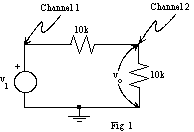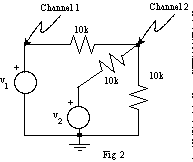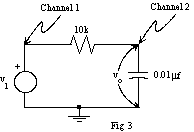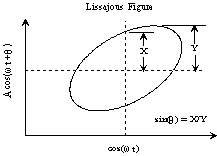EE 212 Lab

Lab 2: Sine Waves and the Oscilloscope

The purpose of this lab is become acquainted with the operation of an oscilloscope, function generator, and AC function of your multimeter by investigating the sinusoidal responses of simple RC circuits.

Laboratory Procedure:

1. The Oscilloscope as a Voltmeter

Turn on the oscilloscope, display the trace for Channel (Ch) 1, and check trigger settings (trigger menu => edge, rising (slope), CH1 (source), auto (mode), DC (coupling)). Apply 0V then 5V to Ch 1 and compare the measured voltages to multimeter readings. More accurate measurements may be obtained by using cursors (cursor => voltage or time (type), CH1 or CH2 (source), then move vertical or horizontal position knobs) or the measure mode (top menu button followed by selecting time or voltage measurements).

2. Sine Waves on the Oscilloscope

a) Apply a small amplitude (less than 5V), 1kHz sine wave from your function generator to Ch 1. Adjust the trigger controls to get a stable waveform display. Experiment with the trigger level, slope, and other controls. Finish with the display being triggered as the waveform crosses zero while rising.

b) Experiment with the time base control. Adjust the sine wave frequency on the frequency generator to be exactly 1kHz. Use the cursors to measure the period. What frequency is displayed on the oscilloscope? Is the reading what you expect? Check the period of ten cycles.

c) Adjust the amplitude of the sine wave to be 1V peak-to-peak (p-p). Measure the amplitude of the sine wave using the oscilloscope. Measure the amplitude with the AC Volts function of the multimeter. How should and how do these numbers compare?

3. Voltage Divider and Superposition

a) Consider the circuit in figure 1.

• Wire up the circuit shown in figure 1 where v1 produces a 5V p-p 1kHz sine wave.
• Display v1 on Ch 1 and output of this voltage divider, vo, on Ch 2. Compare the voltages and phases. Repeat at 10kHz.b) Consider the circuit in figure 2 where a source and resistor have been added to the circuit shown above in figure 1.

• Let v2 be a 5V DC source and use superposition to analytically solve for the output voltage, vo (labeled Channel 2).
• Wire up the circuit shown in figure 2 with v2 a 5V DC source.
• Using the oscilloscope input select control, ground each input to see where 0V DC is on the scope. Adjust the trace positions so that 0V lies on the center graticule and measure vo. Is vo what you expected it to be?
• Connect v2 to ground instead of 5V, and record how the vo waveform changes. Reconnect v2 to 5V and record the average value of vo as you slowly decrease v1 to 0V.
• Return v1 to a 5V p-p sine wave and AC couple the scope inputs. Notice that the DC level is removed when the scope input is AC coupled. This is because a capacitor has been connected in series with the input.4. Phase Measurements

a) Consider the RC circuit shown in figure 3.

• Wire up the RC circuit shown in figure 3 with v1 a 1kHz 5V p-p sine wave.
• Observe the input v1 and output vo on the two channels, and compare their amplitudes and phases.
• Measure R with an ohmmeter, and calculate C from the ratio of amplitudes and from the phase shift. Compare your calculated values of C with the value labeled on the capacitor. Repeat at several frequencies including 100Hz and 10kHz.  What is the maximum phase shift you can get?
• Use the XY mode of the oscilloscope (Display => XY (format)) to display a Lissajous figure as shown in figure 4. Determine the phase angle between v1 and vo from this display.5. Summary

Before you go, make sure you understand the following concepts about the oscilloscope:

• Horizontal controls: Time per division,  trigger level, trigger slope, auto vs. normal sweep.
• Vertical controls: Volts per division, fine vs. course scaling, AC vs. DC coupling.
• XY Mode: Getting into and out of XY mode.
• Measurements: Voltage measurement, phase measurement via time shift, phase measurement in XY mode using Lissajous figure, use of cursors.
• Storage mode.Fig. 4